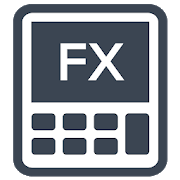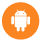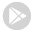Additionally, paste this code immediately after the opening tag:

See whats happening!## Forex Calculators

Essential Calculators for Forex Traders Forex Calculators include: +Position Size Calculator +Stop Lo...

Free### Store review

Forex Calculators include:
+Position Size Calculator
+Stop Loss & Take Profit Calculator
+Risk Reward Calculator
+Margin Calculator
+Pip Value Calculator
+Fibonacci Calculator
+Pivot Points Calculator

Risk management consider to be one of the most important skills in Forex trading. Forex Calculators provide you the necessary tools to develop your risk management skills for Forex traders.

Proper position sizing is the key to managing risk in trading Forex. Position Size Calculator help you calculate the amount of units/lots to put on a single trade based on your risk percentage/amount and stop loss pips/price.

Stop Loss & Take Profit Calculator help you calculate the appropriate stop loss price and take profit price according your position size, risk amount/pips, and risk reward ratio.

Pip Value Calculator help you calculate the single pip value in your account currency based on position size and pip amount.

Margin Calculator help you calculate margin requirements of a trade position based on the position size and the account leverage.

Fibonacci Calculator help you calculate the key levels of Fibonacci retracement and Fibonacci extensions by the input of high and low price.

Pivot Point Calculator help you calculate the support and resistance levels based on varies Pivot Point calculation methods.

All Forex calculations are based on real time market price. Custom price option is also available in case you want to use your own price for calculation. Forex Calculators has included most of the commonly trade currency pairs in the Forex market.

If you have any questions or feedback please feel free to write in the comments or contact me through email.

Store rating

4

out of

1065 reviews

Last update

Feb. 25, 2020